﻿ 电磁场下Pre-BO¨ tzinger Complex神经元的放电活动研究 Discharge Pattern of Neuron in the Pre-BO¨ tzinger Complex under Magnetic Field

Dynamical Systems and Control
Vol.07 No.03(2018), Article ID:24880,7 pages
10.12677/DSC.2018.73016

Discharge Pattern of Neuron in the Pre-Bötzinger Complex under Magnetic Field

Qinyu Cao*, Fen Ma, Wangjuan Liang

School of Science, North China University of Technology, Beijing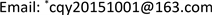Received: Apr. 15th, 2018; accepted: May 9th, 2018; published: May 16th, 2018ABSTRACT

Magnetic field has important effects on the firing activities of neuron. The function of memristor is similar as that of plasticity of synapse. Based on the Butera dynamic model added with memristor, the discharge pattern of pre-Bӧtzinger complex under the magnetic flux is studied. By fast-slow analysis and two-parameter bifurcation analysis, the dynamic mechanisms of the discharge pattern of neurons in the pre-Bӧtzinger complex are studied with the potassium conductance varying.

Keywords:Memristor, Magnetic Flux, Bursting, Fast-Slow Bifurcation Analysis1. 引言

2. 模型

$\left\{\begin{array}{l}\frac{\text{d}v}{\text{d}t}=\left(-{I}_{Nap}-{I}_{Na}-{I}_{K}-{I}_{L}-{I}_{tonic}-{I}_{app}\right)/C\text{\hspace{0.17em}}\text{\hspace{0.17em}}\text{\hspace{0.17em}}\text{\hspace{0.17em}}\text{\hspace{0.17em}}\text{\hspace{0.17em}}\text{\hspace{0.17em}}\text{\hspace{0.17em}}\text{\hspace{0.17em}}\text{\hspace{0.17em}}\text{\hspace{0.17em}}\text{\hspace{0.17em}}\text{\hspace{0.17em}}\text{\hspace{0.17em}}\text{\hspace{0.17em}}\text{\hspace{0.17em}}\text{\hspace{0.17em}}\text{\hspace{0.17em}}\text{\hspace{0.17em}}\text{\hspace{0.17em}}\text{\hspace{0.17em}}\text{\hspace{0.17em}}\text{\hspace{0.17em}}\text{\hspace{0.17em}}\text{\hspace{0.17em}}\text{\hspace{0.17em}}\text{\hspace{0.17em}}\text{\hspace{0.17em}}\text{\hspace{0.17em}}\text{\hspace{0.17em}}\text{\hspace{0.17em}}\text{\hspace{0.17em}}\text{\hspace{0.17em}}\text{ }\text{\hspace{0.17em}}\text{\hspace{0.17em}}\text{\hspace{0.17em}}\text{\hspace{0.17em}}\text{\hspace{0.17em}}\text{\hspace{0.17em}}\text{\hspace{0.17em}}\text{ }\text{\hspace{0.17em}}\text{\hspace{0.17em}}\text{\hspace{0.17em}}\text{\hspace{0.17em}}\left(1\right)\\ \frac{\text{d}h}{\text{d}t}=\epsilon \left({h}_{\infty }\left(v\right)-h\right)/{\tau }_{h}\left(v\right)\text{\hspace{0.17em}}\text{\hspace{0.17em}}\text{\hspace{0.17em}}\text{\hspace{0.17em}}\text{\hspace{0.17em}}\text{\hspace{0.17em}}\text{\hspace{0.17em}}\text{\hspace{0.17em}}\text{\hspace{0.17em}}\text{\hspace{0.17em}}\text{\hspace{0.17em}}\text{\hspace{0.17em}}\text{\hspace{0.17em}}\text{\hspace{0.17em}}\text{\hspace{0.17em}}\text{\hspace{0.17em}}\text{\hspace{0.17em}}\text{\hspace{0.17em}}\text{\hspace{0.17em}}\text{\hspace{0.17em}}\text{\hspace{0.17em}}\text{\hspace{0.17em}}\text{\hspace{0.17em}}\text{\hspace{0.17em}}\text{\hspace{0.17em}}\text{\hspace{0.17em}}\text{\hspace{0.17em}}\text{\hspace{0.17em}}\text{\hspace{0.17em}}\text{\hspace{0.17em}}\text{\hspace{0.17em}}\text{\hspace{0.17em}}\text{\hspace{0.17em}}\text{\hspace{0.17em}}\text{\hspace{0.17em}}\text{\hspace{0.17em}}\text{\hspace{0.17em}}\text{\hspace{0.17em}}\text{\hspace{0.17em}}\text{\hspace{0.17em}}\text{\hspace{0.17em}}\text{\hspace{0.17em}}\text{\hspace{0.17em}}\text{\hspace{0.17em}}\text{\hspace{0.17em}}\text{\hspace{0.17em}}\text{\hspace{0.17em}}\text{\hspace{0.17em}}\text{\hspace{0.17em}}\text{\hspace{0.17em}}\text{\hspace{0.17em}}\text{\hspace{0.17em}}\text{\hspace{0.17em}}\text{\hspace{0.17em}}\text{ }\text{\hspace{0.17em}}\text{\hspace{0.17em}}\text{\hspace{0.17em}}\text{\hspace{0.17em}}\text{\hspace{0.17em}}\text{\hspace{0.17em}}\text{\hspace{0.17em}}\text{\hspace{0.17em}}\text{\hspace{0.17em}}\text{\hspace{0.17em}}\text{\hspace{0.17em}}\text{\hspace{0.17em}}\text{\hspace{0.17em}}\text{\hspace{0.17em}}\left(2\right)\\ \frac{\text{d}n}{\text{d}t}=\left({n}_{\infty }\left(v\right)-n\right)/{\tau }_{n}\left(v\right)\text{\hspace{0.17em}}\text{\hspace{0.17em}}\text{\hspace{0.17em}}\text{\hspace{0.17em}}\text{\hspace{0.17em}}\text{\hspace{0.17em}}\text{\hspace{0.17em}}\text{\hspace{0.17em}}\text{\hspace{0.17em}}\text{\hspace{0.17em}}\text{\hspace{0.17em}}\text{\hspace{0.17em}}\text{\hspace{0.17em}}\text{\hspace{0.17em}}\text{\hspace{0.17em}}\text{\hspace{0.17em}}\text{\hspace{0.17em}}\text{\hspace{0.17em}}\text{\hspace{0.17em}}\text{\hspace{0.17em}}\text{\hspace{0.17em}}\text{\hspace{0.17em}}\text{\hspace{0.17em}}\text{\hspace{0.17em}}\text{\hspace{0.17em}}\text{\hspace{0.17em}}\text{\hspace{0.17em}}\text{\hspace{0.17em}}\text{\hspace{0.17em}}\text{\hspace{0.17em}}\text{\hspace{0.17em}}\text{\hspace{0.17em}}\text{\hspace{0.17em}}\text{\hspace{0.17em}}\text{\hspace{0.17em}}\text{\hspace{0.17em}}\text{\hspace{0.17em}}\text{\hspace{0.17em}}\text{\hspace{0.17em}}\text{\hspace{0.17em}}\text{\hspace{0.17em}}\text{\hspace{0.17em}}\text{\hspace{0.17em}}\text{\hspace{0.17em}}\text{\hspace{0.17em}}\text{\hspace{0.17em}}\text{\hspace{0.17em}}\text{\hspace{0.17em}}\text{\hspace{0.17em}}\text{\hspace{0.17em}}\text{\hspace{0.17em}}\text{\hspace{0.17em}}\text{\hspace{0.17em}}\text{\hspace{0.17em}}\text{\hspace{0.17em}}\text{\hspace{0.17em}}\text{\hspace{0.17em}}\text{ }\text{\hspace{0.17em}}\text{\hspace{0.17em}}\text{\hspace{0.17em}}\text{\hspace{0.17em}}\text{\hspace{0.17em}}\text{\hspace{0.17em}}\text{\hspace{0.17em}}\text{\hspace{0.17em}}\text{ }\text{\hspace{0.17em}}\text{\hspace{0.17em}}\text{\hspace{0.17em}}\text{\hspace{0.17em}}\text{\hspace{0.17em}}\left(3\right)\\ \frac{\text{d}\phi }{\text{d}t}=f\left(\phi \right)\text{\hspace{0.17em}}\text{\hspace{0.17em}}\text{\hspace{0.17em}}\text{\hspace{0.17em}}\text{\hspace{0.17em}}\text{\hspace{0.17em}}\text{\hspace{0.17em}}\text{\hspace{0.17em}}\text{\hspace{0.17em}}\text{\hspace{0.17em}}\text{\hspace{0.17em}}\text{\hspace{0.17em}}\text{\hspace{0.17em}}\text{\hspace{0.17em}}\text{\hspace{0.17em}}\text{\hspace{0.17em}}\text{\hspace{0.17em}}\text{\hspace{0.17em}}\text{\hspace{0.17em}}\text{\hspace{0.17em}}\text{\hspace{0.17em}}\text{\hspace{0.17em}}\text{\hspace{0.17em}}\text{\hspace{0.17em}}\text{\hspace{0.17em}}\text{\hspace{0.17em}}\text{\hspace{0.17em}}\text{\hspace{0.17em}}\text{\hspace{0.17em}}\text{\hspace{0.17em}}\text{\hspace{0.17em}}\text{\hspace{0.17em}}\text{\hspace{0.17em}}\text{\hspace{0.17em}}\text{\hspace{0.17em}}\text{\hspace{0.17em}}\text{\hspace{0.17em}}\text{\hspace{0.17em}}\text{\hspace{0.17em}}\text{\hspace{0.17em}}\text{\hspace{0.17em}}\text{\hspace{0.17em}}\text{\hspace{0.17em}}\text{\hspace{0.17em}}\text{\hspace{0.17em}}\text{\hspace{0.17em}}\text{\hspace{0.17em}}\text{\hspace{0.17em}}\text{\hspace{0.17em}}\text{\hspace{0.17em}}\text{\hspace{0.17em}}\text{\hspace{0.17em}}\text{\hspace{0.17em}}\text{\hspace{0.17em}}\text{\hspace{0.17em}}\text{\hspace{0.17em}}\text{\hspace{0.17em}}\text{\hspace{0.17em}}\text{\hspace{0.17em}}\text{\hspace{0.17em}}\text{\hspace{0.17em}}\text{\hspace{0.17em}}\text{\hspace{0.17em}}\text{\hspace{0.17em}}\text{\hspace{0.17em}}\text{\hspace{0.17em}}\text{\hspace{0.17em}}\text{\hspace{0.17em}}\text{\hspace{0.17em}}\text{\hspace{0.17em}}\text{\hspace{0.17em}}\text{\hspace{0.17em}}\text{\hspace{0.17em}}\text{\hspace{0.17em}}\text{\hspace{0.17em}}\text{\hspace{0.17em}}\text{\hspace{0.17em}}\text{ }\text{\hspace{0.17em}}\text{\hspace{0.17em}}\text{ }\text{\hspace{0.17em}}\text{\hspace{0.17em}}\text{\hspace{0.17em}}\text{\hspace{0.17em}}\text{\hspace{0.17em}}\text{\hspace{0.17em}}\text{\hspace{0.17em}}\left(4\right)\\ \frac{\text{d}q\left(\phi \right)}{\text{d}\phi }=\rho \left(\phi \right)\text{\hspace{0.17em}}\text{\hspace{0.17em}}\text{\hspace{0.17em}}\text{\hspace{0.17em}}\text{\hspace{0.17em}}\text{\hspace{0.17em}}\text{\hspace{0.17em}}\text{\hspace{0.17em}}\text{\hspace{0.17em}}\text{\hspace{0.17em}}\text{\hspace{0.17em}}\text{\hspace{0.17em}}\text{\hspace{0.17em}}\text{\hspace{0.17em}}\text{\hspace{0.17em}}\text{\hspace{0.17em}}\text{\hspace{0.17em}}\text{\hspace{0.17em}}\text{\hspace{0.17em}}\text{\hspace{0.17em}}\text{\hspace{0.17em}}\text{\hspace{0.17em}}\text{\hspace{0.17em}}\text{\hspace{0.17em}}\text{\hspace{0.17em}}\text{\hspace{0.17em}}\text{\hspace{0.17em}}\text{\hspace{0.17em}}\text{\hspace{0.17em}}\text{\hspace{0.17em}}\text{\hspace{0.17em}}\text{\hspace{0.17em}}\text{\hspace{0.17em}}\text{\hspace{0.17em}}\text{\hspace{0.17em}}\text{\hspace{0.17em}}\text{\hspace{0.17em}}\text{\hspace{0.17em}}\text{\hspace{0.17em}}\text{\hspace{0.17em}}\text{\hspace{0.17em}}\text{\hspace{0.17em}}\text{\hspace{0.17em}}\text{\hspace{0.17em}}\text{\hspace{0.17em}}\text{\hspace{0.17em}}\text{\hspace{0.17em}}\text{\hspace{0.17em}}\text{\hspace{0.17em}}\text{\hspace{0.17em}}\text{\hspace{0.17em}}\text{\hspace{0.17em}}\text{\hspace{0.17em}}\text{\hspace{0.17em}}\text{\hspace{0.17em}}\text{\hspace{0.17em}}\text{\hspace{0.17em}}\text{\hspace{0.17em}}\text{\hspace{0.17em}}\text{\hspace{0.17em}}\text{\hspace{0.17em}}\text{\hspace{0.17em}}\text{\hspace{0.17em}}\text{\hspace{0.17em}}\text{\hspace{0.17em}}\text{\hspace{0.17em}}\text{\hspace{0.17em}}\text{\hspace{0.17em}}\text{\hspace{0.17em}}\text{\hspace{0.17em}}\text{\hspace{0.17em}}\text{\hspace{0.17em}}\text{\hspace{0.17em}}\text{ }\text{\hspace{0.17em}}\text{\hspace{0.17em}}\text{\hspace{0.17em}}\text{\hspace{0.17em}}\text{\hspace{0.17em}}\text{\hspace{0.17em}}\text{\hspace{0.17em}}\text{\hspace{0.17em}}\text{\hspace{0.17em}}\left(5\right)\end{array}$

${I}_{Nap}={g}_{Nap}{m}_{p,\infty }\left(v\right)h\left(v-{E}_{Na}\right)$${I}_{Na}={g}_{Na}{m}_{\infty }^{3}\left(v\right)\left(1-n\right)\left(v-{E}_{Na}\right)$

${I}_{K}={g}_{K}{n}^{4}\left(v\right)\left(v-{E}_{k}\right)$${I}_{L}={g}_{L}\left(v-{E}_{L}\right)$${I}_{tonic}={g}_{tonic\text{-}e}\left(v-{E}_{syn\text{-}e}\right)$

${I}_{app}={k}_{1}\ast v\ast \rho \left(\phi \right)$$f\left(\phi \right)=v-{k}_{2}\ast \phi$

$\rho \left(\phi \right)$ 是指磁通控制忆阻器，用来描述磁通量φ与v之间的关系，其表达式为： $\rho \left(\phi \right)=\alpha +3\beta {\phi }^{2}$ ，其中 $\alpha ,\beta$ 是给定的参数值。参数 ${k}_{1}$ 对膜电位上的电流起到调节作用， ${k}_{2}$ 用来抑制磁通量的增加。其它函数的具体表达式以及参数的取值详见附录。

3. 神经元放电行为转迁的分析

${g}_{K}=5$ 时，在不考虑和考虑磁通量并添加磁流忆阻器的条件下，神经元的放电模式分别如图1(a)，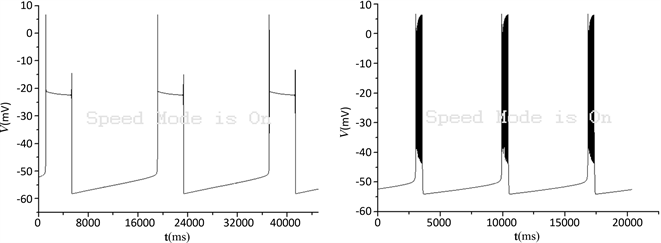(a) (b)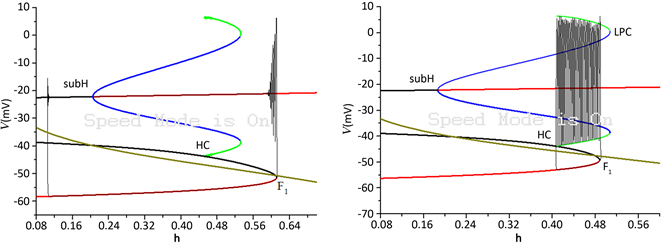(c) (d)

Figure 1. Neuronal discharge patterns and fast/slow bifurcation analysis with ${g}_{K}=5$ . (a) Membrane potential under conditions no magnetic flow with ${I}_{app}=0,\text{\hspace{0.17em}}f\left(\phi \right)=0,\text{\hspace{0.17em}}\rho \left(\phi \right)=0$ ; (b) The time series of membrane potential under magnetic flow with ${k}_{1}=0.05,\text{\hspace{0.17em}}{k}_{2}=2,\text{\hspace{0.17em}}\alpha =2,\text{\hspace{0.17em}}\beta =0.0005$ ; (c) Fast/slow bifurcation analysis of bursting under the same parameter set as that of (a); (d) fast/slow bifurcation analysis of bursting under the same parameter set as that of (b)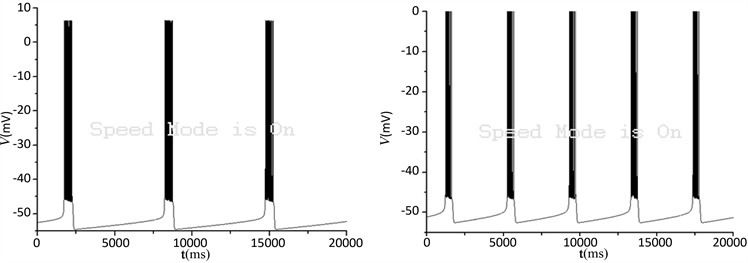(a) (b)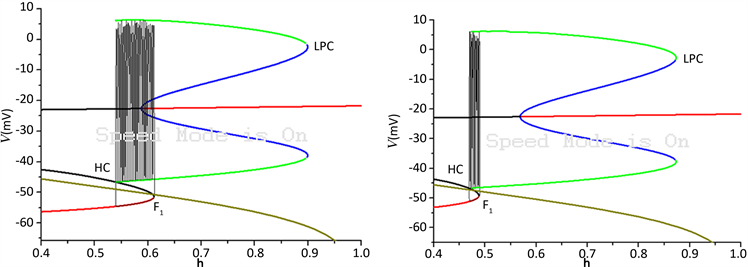(c) (d)

Figure 2. Neuronal discharge patterns and fast/slow bifurcation analysis with ${g}_{K}=8.5$ . (a) Membrane potentialunder conditions no magnetic flow with ${I}_{app}=0,\text{\hspace{0.17em}}f\left(\phi \right)=0,\text{\hspace{0.17em}}\rho \left(\phi \right)=0$ ; (b) The time series of membrane potential under magnetic flow with ${k}_{1}=0.05,\text{\hspace{0.17em}}{k}_{2}=2,\text{\hspace{0.17em}}\alpha =2,\text{\hspace{0.17em}}\beta =0.0005$ ; (c) Fast/slow bifurcation analysis of bursting under the same parameter set as that of (a); (d) fast/slow bifurcation analysis of bursting under the same parameter set as that of (b)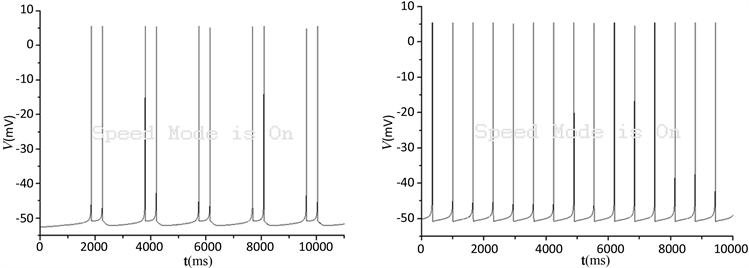(a) (b)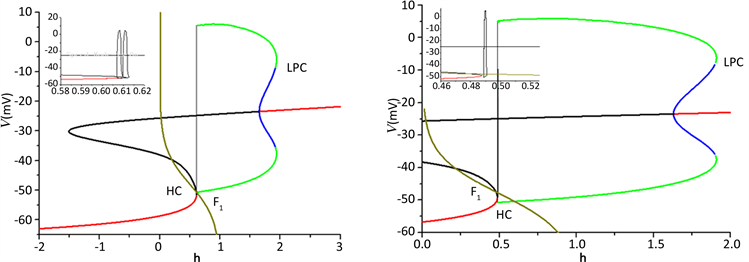(c) (d)

Figure 3. Neuronal discharge patterns and fast/slow bifurcation analysis with ${g}_{K}=20$ . (a) Membrane potentialunder conditions no magnetic flow with ${I}_{app}=0,\text{\hspace{0.17em}}f\left(\phi \right)=0,\text{\hspace{0.17em}}\rho \left(\phi \right)=0$ ; (b) The time series of membrane potential under magnetic flow with ${k}_{1}=0.05,\text{\hspace{0.17em}}{k}_{2}=2,\text{\hspace{0.17em}}\alpha =2,\text{\hspace{0.17em}}\beta =0.0005$ ; (c) Fast/slow bifurcation analysis of bursting under the same parameter set as that of (a); (d) fast/slow bifurcation analysis of spiking under the same parameter set as that of (b)

“fold/homoclinic”型簇放电，如图2(c)和图2(d)所示。

${g}_{K}=20$ 时，磁流作用使得神经元的放电模式由周期为2的簇放电转变为周期为1的峰放电，如图3(a)和图3(b)所示，对应的快慢分析分别如图3(c)和图3(d)所示。磁流作用使得神经元的放电周期变小，频率增大。同时，磁流作用使得双稳区域消失，此时只存在稳定的极限环吸引子(图3(d))，即神经元的放电模式为峰放电(图3(b))。也即，磁流作用使得神经元的放电模式由簇放电转变为峰放电。

4. 双参数分岔分析

5. 结论(a) (b)

Figure 4. Two parameter bifurcation analysis of the fast subsystem in $\left(h,{g}_{K}\right)$ plane. (a) ${I}_{app}=0,\text{\hspace{0.17em}}f\left(\phi \right)=0,\text{\hspace{0.17em}}\rho \left(\phi \right)=0$ , discharge patterns of neuron are not affected by the magnetic flow; (b) ${k}_{1}=0.05,\text{\hspace{0.17em}}{k}_{2}=2,\text{\hspace{0.17em}}\alpha =2,\text{\hspace{0.17em}}\beta =0.0005$ , under the conditions of magnetic flow

Discharge Pattern of Neuron in the Pre-BO¨ tzinger Complex under Magnetic Field[J]. 动力系统与控制, 2018, 07(03): 147-153. https://doi.org/10.12677/DSC.2018.73016

1. 1. Smith, J.C., Ellenberger, H.H., Ballanyi, K., Richter, D.W. and Feldman, J.L. (1991) Pre-Bӧtzinger Complex: A Brain Stem Region That May Generate Respiratory Rhythm in Mammals. Science, 254, 726-729.
https://doi.org/10.1126/science.1683005

2. 2. 刘义, 刘深泉. Pre-Bӧtzinger中间神经元模型的动力学分析[J]. 动力学与控制学报, 2011, 9(3): 257-262.

3. 3. Gaiteri, C. and Rubin, J.E. (2011) The Interaction of Intrinsic Dynamics and Network Topology in Determining Network Burst Synchrony. Frontiers in Computational Neuroscience, 5, Article 10.

4. 4. 文治洪, 胡三觉, 董秀珍, 等. 噪声引起神经元动力学特征的改变[J]. 医学争鸣, 2004, 25(10): 948-949.

5. 5. Ren, G., Xu, Y. and Wang, C. (2017) Synchronization Behavior of Coupled Neuron Circuits Composed of Memristors. Nonlinear Dynamics, 88, 893-901.
https://doi.org/10.1007/s11071-016-3283-2

6. 6. Vaidyanathan, S., Volos, C.K., Kyprianidis, I.M., et al. (2015) Memristor: A New Concept in Synchronization of Coupled Neuromorphic Circuits. Journal of Engineering Science & Technology Review, 8, 157-173.

7. 7. 李佳佳, 吴莹, 独盟盟, 等. 电磁辐射诱发神经元放电节律转迁的动力学行为研究[J]. 物理学报, 2015, 64(3): 214-220.

8. 8. 李洪明, 张素丽. 恒电流刺激下神经元Chay模型的Hopf分岔分析[J]. 太原理工大学学报, 2013, 44(1): 123-126.

9. 9. Stuchly, M.A. and Dawson, T.W. (2000) Interaction of Low-Frequency Electric and Magnetic Fields with the Human Body. Proceedings of the IEEE, 88, 643-664.
https://doi.org/10.1109/5.849161

10. 10. Wertheimer, N. and Leeper, E. (1979) Electrical Wiring Configurations and Childhood Cancer. American Journal of Epidemiology, 109, 273-284.
https://doi.org/10.1093/oxfordjournals.aje.a112681

11. 11. Butera, R.J., Rinzel, J. and Smith, J.C. (1999) Models of Respiratory Rhythm Generation in the Pre-Bӧtzinger Complex. I. Bursting Pacemaker Neurons. Journal of Neurophysiology, 82, 382-397.
https://doi.org/10.1152/jn.1999.82.1.382